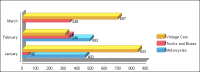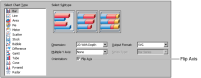Transposing chart axes
Transposing the axes of a chart plots categories on the vertical axis and values on the horizontal axis. Figure 21‑21 shows a bar chart using transposed axes.Figure 21‑21 Bar chart with transposed axes
Two-dimensional charts and charts using depth support transposing axes. Three‑dimensional charts do not support transposing axes. To transpose axes, navigate to the Select Chart Type page, then select Flip Axis, as shown in Figure 21‑22.Figure 21‑22 Transposing the axes of a chart